# Casio Scientific Calculator FX-570MS2 Second Edition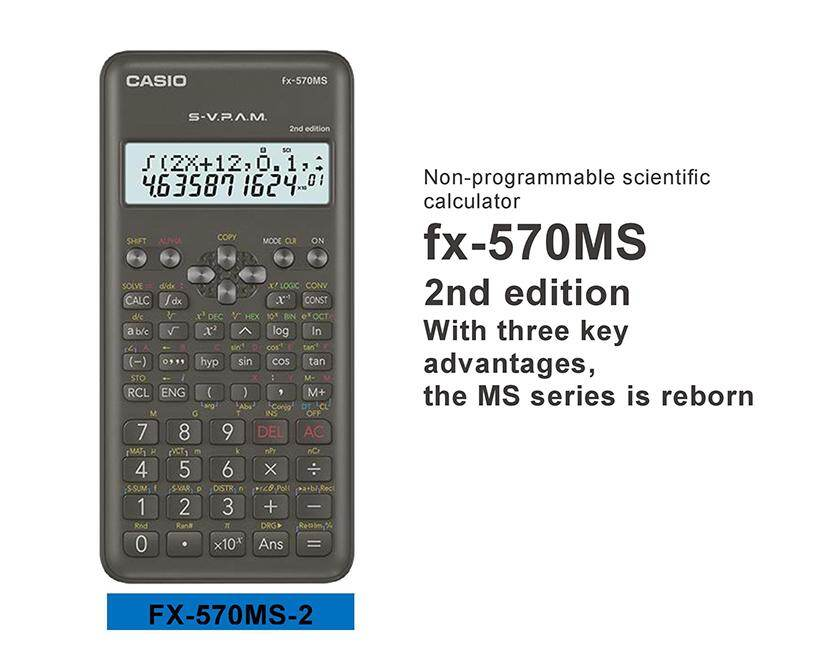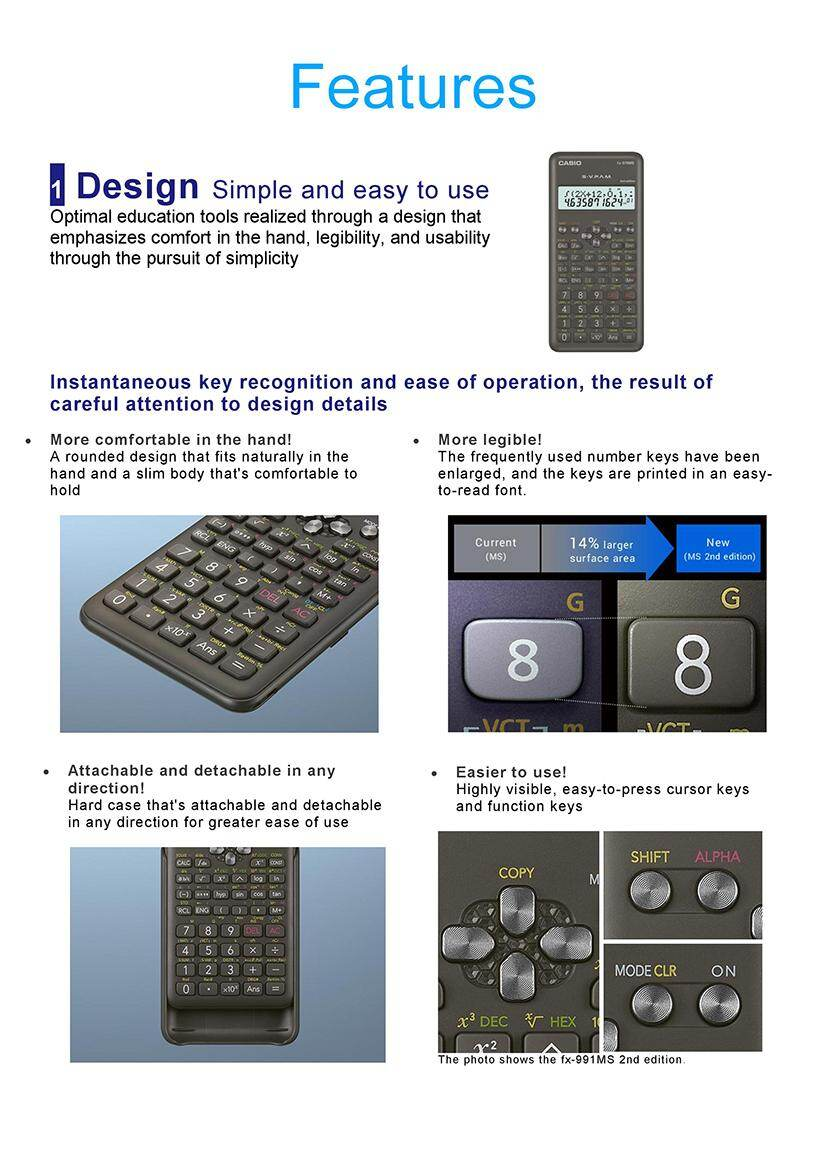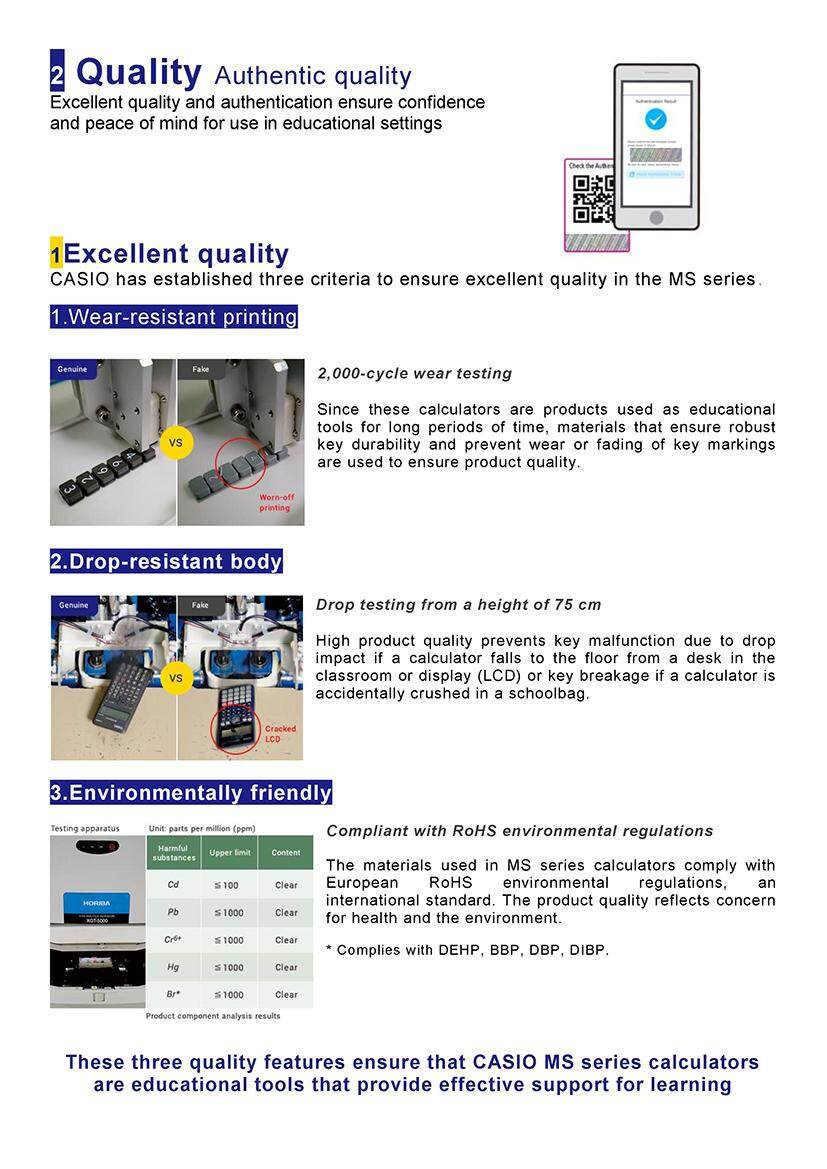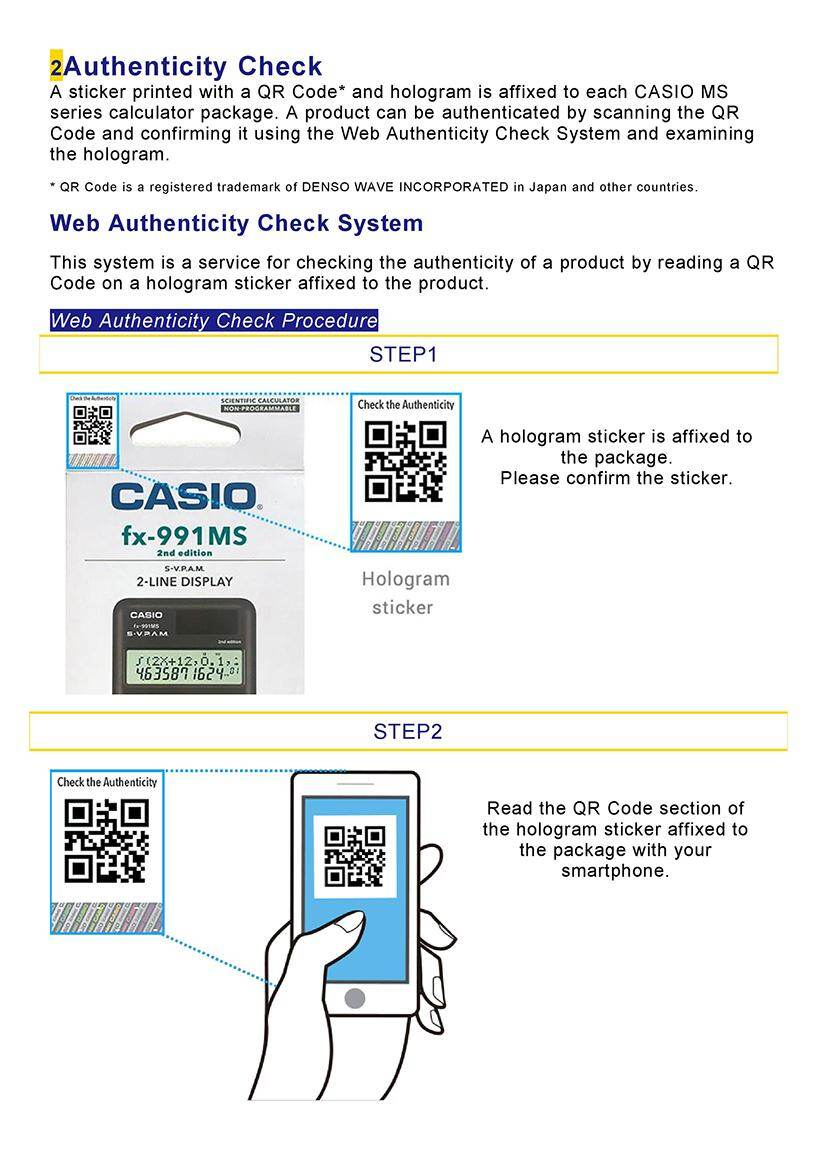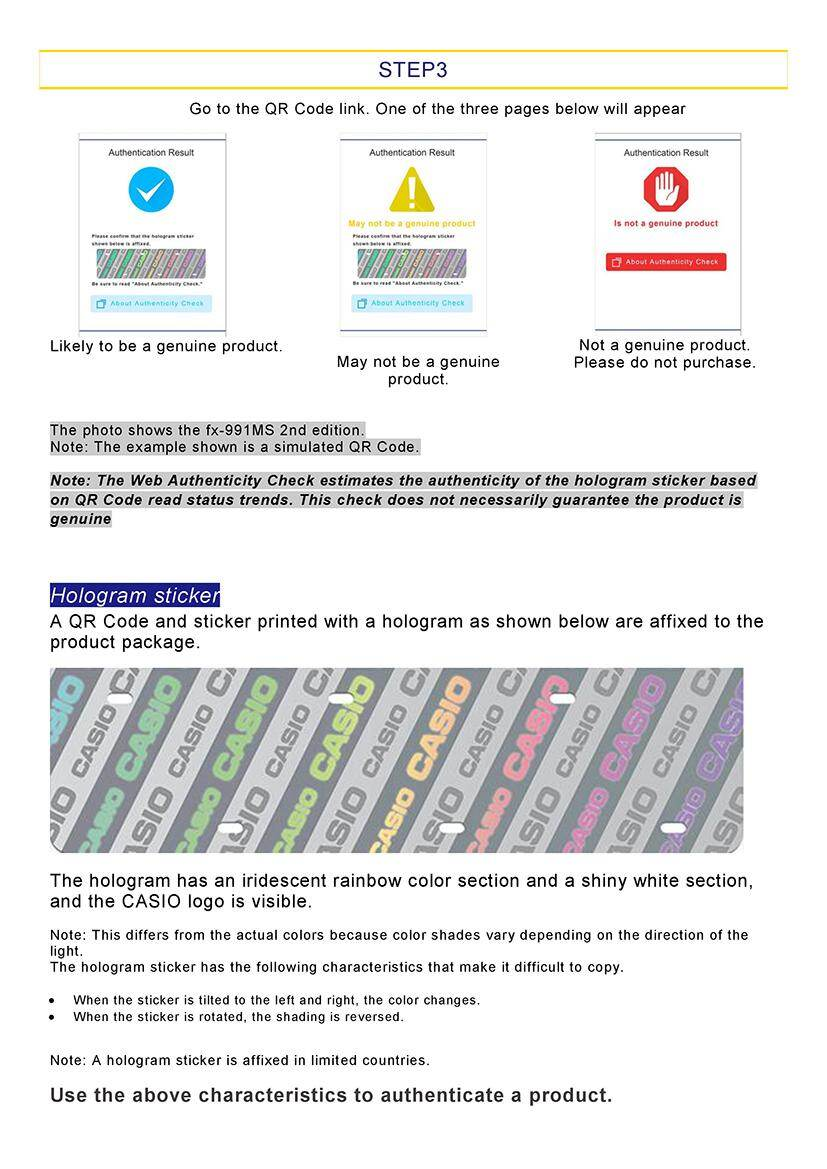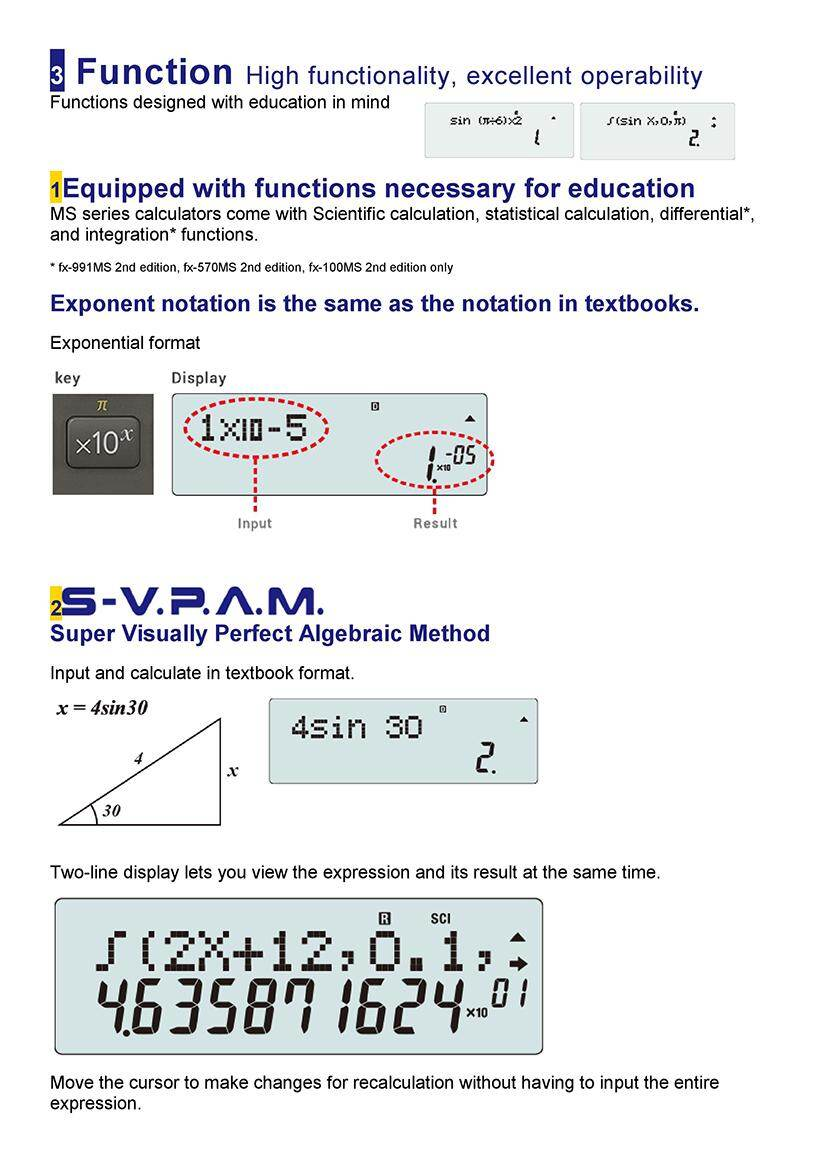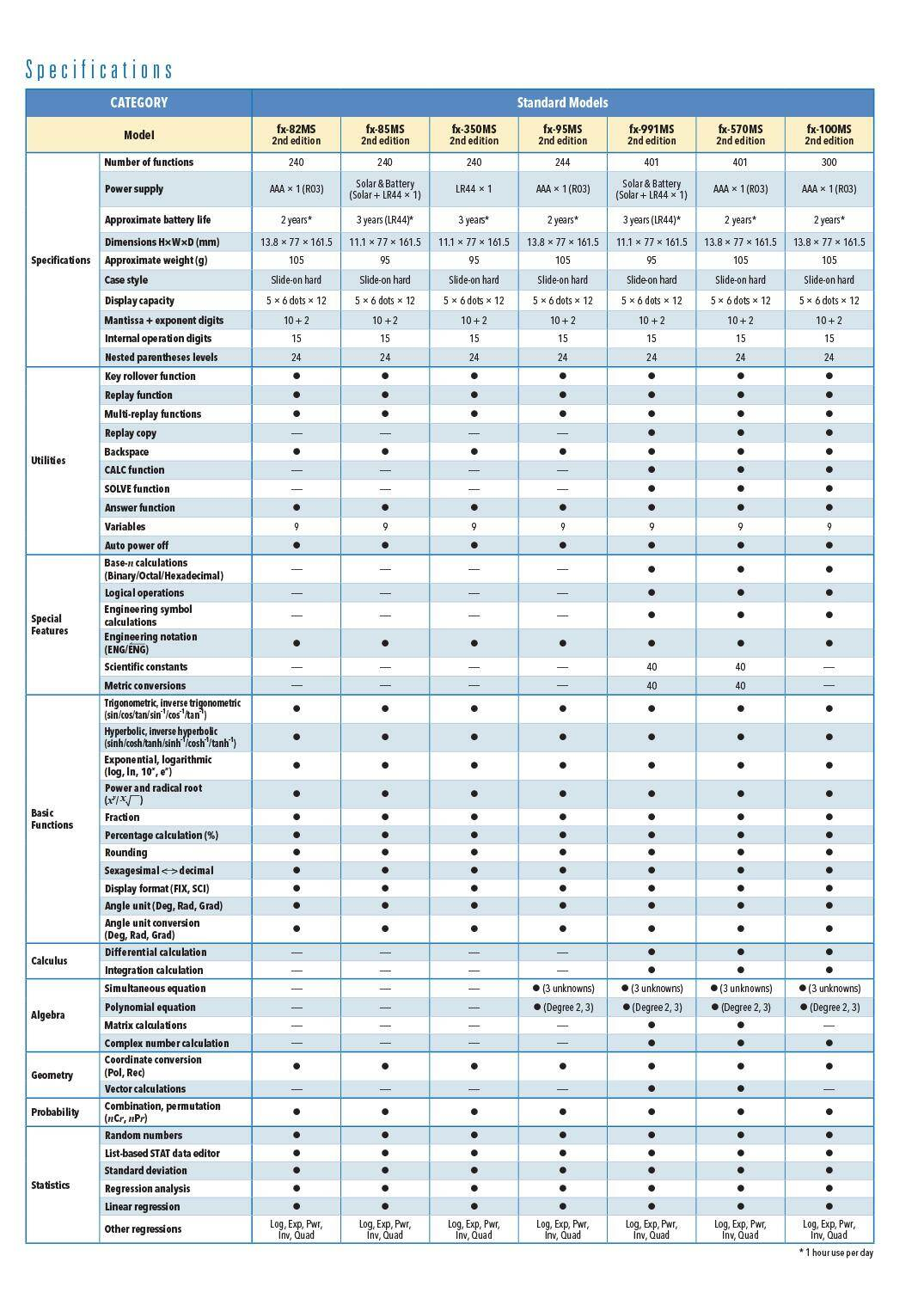Specifications:

• 401 Functions

• S-V.P.A.M. ~super visually perfect algebraic method

• Fraction Calculations

• Combination and permutation

• Statistics (STAT-data editor, Standard deviation, Regression analysis)

• 9 Variables

• Multi-replay

• Equation calculations, Vector calculations, Differential calculations, SOLVE function

• Integration calculations

• Base-n calculations/ conversions

• Complex number calculations

• Logical operations

• Matrix calculations

• Engineering symbol calculations

• 40 Scientific calculations

• 40 Matrix conversions (20 conversion pairs)

• CALC memory

• 2-Line Display

• 10+2 Digits

• Dot Matrix

• Plastic Keys

• Comes with slide-on hard case

• AAA Battery

• 1-year warranty

• 12.7mm x 78mm x 154.5mm

(H x W x D)

#### Write a review

Note: HTML is not translated!